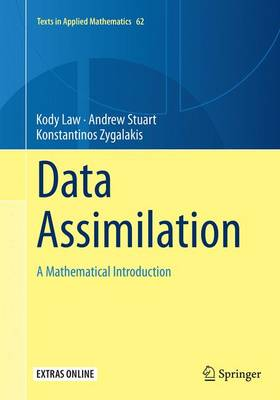•Data Assimilation: A Mathematical Introduction - Texts in Applied Mathematics 62 (Paperback)

(author), (author), (author)
£44.99
Paperback 242 Pages / Published: 22/10/2016
• We can order this

Usually dispatched within 3 weeks

This book provides a systematic treatment of the mathematical underpinnings of work in data assimilation, covering both theoretical and computational approaches. Specifically the authors develop a unified mathematical framework in which a Bayesian formulation of the problem provides the bedrock for the derivation, development and analysis of algorithms; the many examples used in the text, together with the algorithms which are introduced and discussed, are all illustrated by the MATLAB software detailed in the book and made freely available online.

The book is organized into nine chapters: the first contains a brief introduction to the mathematical tools around which the material is organized; the next four are concerned with discrete time dynamical systems and discrete time data; the last four are concerned with continuous time dynamical systems and continuous time data and are organized analogously to the corresponding discrete time chapters.

This book is aimed at mathematical researchers interested in a systematic development of this interdisciplinary field, and at researchers from the geosciences, and a variety of other scientific fields, who use tools from data assimilation to combine data with time-dependent models. The numerous examples and illustrations make understanding of the theoretical underpinnings of data assimilation accessible. Furthermore, the examples, exercises and MATLAB software, make the book suitable for students in applied mathematics, either through a lecture course, or through self-study.

Publisher: Springer International Publishing AG
ISBN: 9783319366876
Number of pages: 242
Weight: 5533 g
Dimensions: 254 x 178 mm
Edition: Softcover reprint of the original 1st ed. 201

MEDIA REVIEWS

"The mathematical style of the book is accessible to post-graduate students and combines formal mathematics with intuitive arguments and summaries of higher level results. ... the book is a good guide on dynamic data assimilation. ... the book suitable as a reference book for modelling on coordinates, whenever the sample space has a Euclidean vector space structure." (Vera Pawlowsky-Glahn, zbMATH 1353.60002, 2017)

"This book provides a Bayesian perspective of data assimilation, with a focus on smoothing and filtering problems with generic dynamical models. ... The authors also provide many numerical results, focusing on simple models that help the reader easily grasp the important properties of the underlying algorithms. In my opinion, this book is well suited to a graduate level course on data assimilation for applied mathematicians." (David T. B. Kelly, Mathematical Reviews, December, 2016)

"The authors have used a collection of dynamical systems examples throughout the book. ... Exercises are also given at the end of each chapter. The first half of this book would be very suitable as a graduate level textbook and concise reference on discrete time approaches to the data assimilation problem from a Bayesian point of view. The second half of the book ... will primarily be of interest to researchers working in this area." (Brian Borchers, MAA Reviews, maa.org, May, 2016)